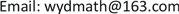1. 引言

2. 存在的问题

1) 缺乏微分几何与其他课程的联系

2) 缺乏抽象理论与具体实践的结合

3) 计算能力和几何直观能力不足

4) 几何课程与课时显少

3. 一些教学探索

3.1. 加强知识交叉及新理论运用

Ι Ι = L d u 2 + 2 M d u d v + N d v 2 = ( d u , d v ) [ L M M N ] ( d u d v )

∬ g ( x , y ) d x d y = ∬ g ( x ( u , v ) , y ( u , v ) ) | ∂ ( x , y ) ∂ ( u , v ) | d u d v

3.2. 加强实践及计算能力

3.3. 加强几何直观

3.4. 增加课时与课程内容